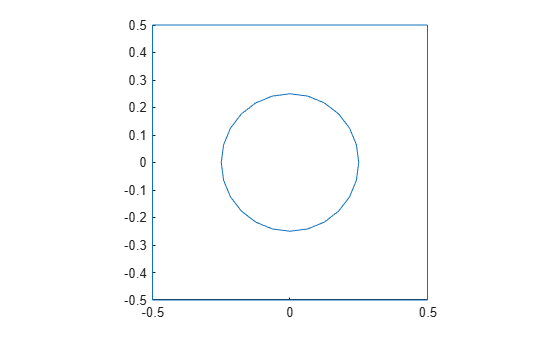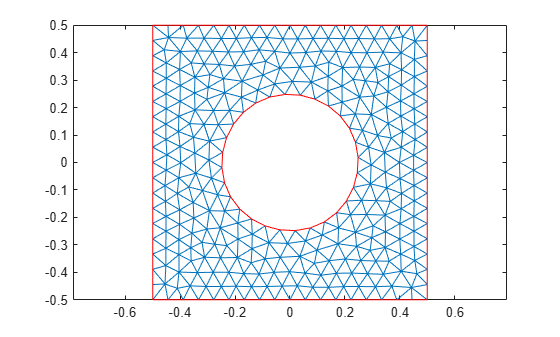# Geometry from `polyshape`

This example shows how to create a polygonal geometry using the MATLAB `polyshape` function. Then use the triangulated representation of the geometry as an input mesh for the `geometryFromMesh` function.

Create and plot a `polyshape` object of a square with a hole.

```t = pi/12:pi/12:2*pi; pgon = polyshape({[-0.5 -0.5 0.5 0.5], 0.25*cos(t)}, ... {[0.5 -0.5 -0.5 0.5], 0.25*sin(t)})```
```pgon = polyshape with properties: Vertices: [29x2 double] NumRegions: 1 NumHoles: 1 ```
```plot(pgon) axis equal```Create a triangulation representation of this object.

`tr = triangulation(pgon);`

Create a PDE model.

`model = createpde;`

With the triangulation data as a mesh, use the `geometryFromMesh` function to create a geometry. Plot the geometry.

```tnodes = tr.Points'; telements = tr.ConnectivityList'; geometryFromMesh(model,tnodes,telements); pdegplot(model)```Plot the mesh.

```figure pdemesh(model)```Because the triangulation data resulted in a low-quality mesh, generate a new finer mesh for further analysis.

`generateMesh(model)`
```ans = FEMesh with properties: Nodes: [2x1259 double] Elements: [6x579 double] MaxElementSize: 0.0566 MinElementSize: 0.0283 MeshGradation: 1.5000 GeometricOrder: 'quadratic' ```

Plot the mesh.

```figure pdemesh(model)```## SupportGet trial now